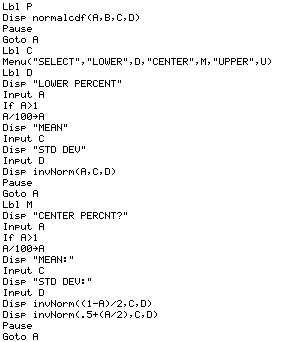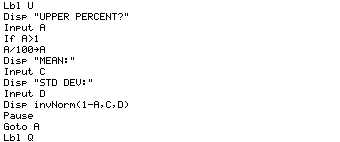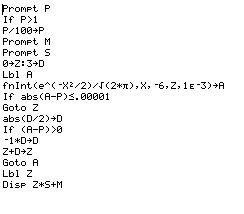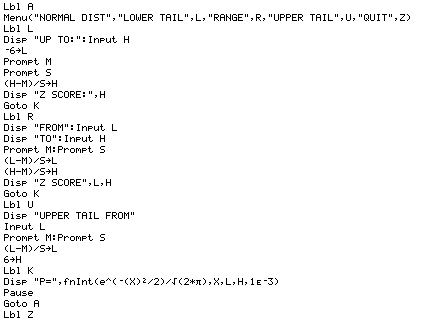# Normal Distribution Programs

## NORM (for the TI-83/TI-83-Plus)

If you have a TI-83, and fear you may forget how to use the built in normal distribution functions, the program NORM organizes them so you can either look at the probability of a z-score, or a z-score for a probability## INVNORM82 (for the TI-82)

For calculators without the built-in distribution functions, the fnInt can be used effectively. invnorm will find a z-score based on a probability.## NORMCDF82 (for the TI-82)

For calculators without the built-in distribution functions, the fnInt can be used effectively. NORMCDF82 will compute a probability for upper and lower tails, or a range. Note you can sacrifice speed for accuracy (and vice versa) by manipulating the tolerance from 1E-3 to 1E-6.## Other Ideas

You can also produce your own table (which will take about 10 minutes to make), and then write a simple lookup program that uses it. For instance, If you produce the table from -3.00 to +3.00, stepping in .01 increments:Then the cumulative probability for -1.64 would be in lGAUSS( 301+ (-1.64)*100 ) That is (301+x*100). You can easily write another program to find the invNorm value as well. When you found the correct amount in the list's contents the index (location in the list) can be converted to a z-score by dividing by 100 and subtracting 301 z = (-301 + i)/100) (so the 70th entry (=.01044) would correspond with z=(-301+70)/100=-2.31 )

## Other Calc Programs and Data

 Mac PC TI-82 Data ti82data.hqx ti82data.zip TI-82 Programs ti82prgm.hqx ti82prgm.zip TI-83 Data ti83data.hqx ti83data.zip TI-83 Programs ti83prgm.hqx ti83prgm.zip TI-85 Data TI-85 Programs